login  home  contents  what's new  discussion  bug reports help  links  subscribe  changes  refresh  edit

## Introduction

This file contains an implementation of rational interpolation, where the data points are element of any integral domain.

## Questions and Outlook

• Maybe this file should be joined with pinterp.spad, where polynomial Lagrange interpolation is implemented. This version parallels the structure of pinterp.spad closely. This also answers comments and questions from wyscc. He remarked
• Abbreviations for a constructor should be limited to 7 letters (not 8). The system occasionally adds the 8th character to a package for internal use.
• Function names begin with a lower case, so RationalInterpolation should be rationalInterpolation, or better, rationalInterpolate.
• Regarding the types I used for the values, wyscc remarked
• If we are doing a rational interpolation, presumably the values are rational, so it does not make sense to require the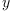-coordinates of inputs be integral. On the other hand, as in the above example, if one uses FRAC INT, problems can arise when this package is combined with other packages that constructs the quotient field of the parameter domain F because Axiom does not like constructing FRAC FRAC INT.

Note however, that the package would rather construct the type FRAC SUP FRAC INT, so this problem should not occur. Moreover, there are situations - for example in the package [mantepse.spad2]?, where we want to interpolate values from an IntegralDomain?. Of course we could first convert them to the quotient field, however, the current approach seems more natural to me.

• Finally, wyscc asked: If p(xx) = interpolate(lx, ly, m, k), what is the purpose of elt(px, qx) = p(qx), the composition of p(xx) and qx, especially when qx is from FRAC UP(xx, F) instead of from just F? and why is this function (the composition) also called interpolate?

I do not really know - apart from a very superficial level: Clearly, the second function was intended to let the user easily plug in values into the interpolated function. I don't find this sensible and I would be happy to change it. Indeed, this would also get rid of the first parameter to RINTERP, which is quite a nuisance.

I think we should agree on a general interface for interpolation algorithms, and mark PINTERP as obsolete. By the way, it seems that RINTERP is faster, too.

• There are probably better ways to implement rational interpolation. Maybe http://www.cs.ucsb.edu/~omer/personal/abstracts/rational.html contains something useful. In particular, in my package [mantepse.spad2]?, in guessRat and guessExpRat I generate interpolating polynomials for all possible degrees of numerator and denominator. The above article contains an algorithm that does this in time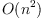, which would be quite nice. Currently, I needoperations for each degree!
• For polynomial interpolation, there seems to be an algorithm that needs only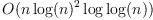operations. It can be found in van zur Gathen's book "Modern computer algebra", chapter 10.
• For those who speak german, http://www.num.math.uni-goettingen.de/schaback/teaching/numath.ps contains quite a bit of information.
• This implementation of rational interpolation neither takes care of unattainable points, nor does it check whether the values of the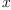-coordinates are all distinct.

)abbrev package RINTERPA RationalInterpolationAlgorithms
++ Description:
++ This package exports rational interpolation algorithms
RationalInterpolationAlgorithms(F, P): Cat == Body   where
F: IntegralDomain
P: UnivariatePolynomialCategory(F)
Cat == with
RationalInterpolation: (List F, List F, NonNegativeInteger,
NonNegativeInteger)
-> Fraction P
+++ We assume that the elements of the first list are all distinct.
+++ If they are not, division by zero might occur.
RationalInterpolation(xlist, ylist, m, k) ==
#xlist ^= #ylist =>
error "Different number of points and values."
#xlist ^= m+k+1 =>
error "wrong number of points"
tempvec: List F := [1 for i in 1..(m+k+1)]
collist: List List F := cons(tempvec,
[(tempvec := [tempvec.i * xlist.i _
for i in 1..(m+k+1)]) _
for j in 1..max(m, k)])
collist := append([collist.j for j in 1..(m+1)], _
[[- collist.j.i * ylist.i for i in 1..(m+k+1)] _
for j in 1..(k+1)])
resspace: List Vector F := nullSpace((transpose matrix collist) _
::Matrix F)
reslist: List List P := _
[[monomial((resspace.1).(i+1), i) for i in 0..m], _
[monomial((resspace.1).(i+m+2), i) for i in 0..k]]
reduce((_+), reslist.1)/reduce((_+), reslist.2)
   Compiling FriCAS source code from file
using old system compiler.
RINTERPA abbreviates package RationalInterpolationAlgorithms
******** Spad syntax error detected ********
The prior line was:
15>         RationalInterpolation(xlist, ylist, m, k) ==
The current line is:
16>             #xlist ^= #ylist =>
The number of valid tokens is 2.
The prior token was #S(TOKEN
:SYMBOL ^
:TYPE KEYWORD
:NONBLANK NIL
:LINE_NUM 16
:CHAR_NUM 19)
The current token is #S(TOKEN
:SYMBOL =
:TYPE KEYWORD
:NONBLANK NIL
:LINE_NUM 16
:CHAR_NUM 20)
The next token is #S(TOKEN
:SYMBOL |#|
:TYPE KEYWORD
:NONBLANK NIL
:LINE_NUM 16
:CHAR_NUM 22)

)abbrev package RINTERP RationalInterpolation
++ Description:
++ This package exports interpolation algorithms
RationalInterpolation(xx, F): Cat == Body   where
xx: Symbol
F:  IntegralDomain
UP  ==> UnivariatePolynomial
SUP ==> SparseUnivariatePolynomial
Cat == with
interpolate: (Fraction UP(xx, F), List F, List F, _
NonNegativeInteger, NonNegativeInteger) _
-> Fraction UP(xx, F)
interpolate: (List F, List F, NonNegativeInteger, NonNegativeInteger) _
-> Fraction SUP F
RIA ==> RationalInterpolationAlgorithms
interpolate(qx, lx, ly, m, k) ==
px := RationalInterpolation(lx, ly, m, k)$RIA(F, UP(xx, F)) elt(px, qx) interpolate(lx, ly, m, k) == RationalInterpolation(lx, ly, m, k)$RIA(F, SUP F)
   Compiling FriCAS source code from file
using old system compiler.
RINTERP abbreviates package RationalInterpolation
------------------------------------------------------------------------
initializing NRLIB RINTERP for RationalInterpolation
compiling into NRLIB RINTERP
processing macro definition RIA ==> RationalInterpolationAlgorithms
compiling exported interpolate : (Fraction UnivariatePolynomial(xx,F),List F,List F,NonNegativeInteger,NonNegativeInteger) -> Fraction UnivariatePolynomial(xx,F)
****** comp fails at level 3 with expression: ******
error in function interpolate
(SEQ
(|:=| |px| | << |
((|Sel| (|RationalInterpolationAlgorithms| F (|UnivariatePolynomial| |xx| F))
|RationalInterpolation|)
|lx| |ly| |m| |k|)
| >> |)
(|exit| 1 (|elt| |px| |qx|)))
****** level 3  ******
$x:= ((Sel (RationalInterpolationAlgorithms F (UnivariatePolynomial xx F)) RationalInterpolation) lx ly m k)$m:= $EmptyMode$f:=
((((|k| # #) (|m| # #) (|ly| # #) (|lx| # #) ...)))
>> Apparent user error:
no modemap for RationalInterpolation with 4 arguments

First we check whether we have the right number of points and values. Clearly the number of points and the number of values must be identical. Note that we want to determine the numerator and denominator polynomials only up to a factor. Thus, we want to determine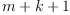coefficients, where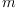is the degree of the polynomial in the numerator and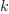is the degree of the polynomial in the denominator.

In fact, we could also leave - for example -unspecified and determine it as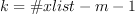: I don't know whether this would be better.

The next step is to set up the matrix. Suppose that our numerator polynomial is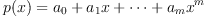and that our denominator polynomial is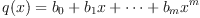. Then we have the following equations, writing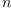for: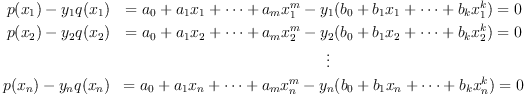This can be written as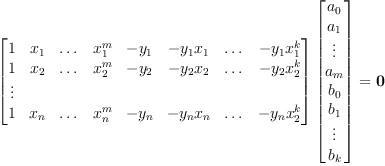We generate this matrix columnwise, then we can solve the system using nullSpace.

Note that it may happen that the system has several solutions. In this case, some of the data points may not be interpolated correctly. However, the solution is often still useful, thus we do not signal an error.

Since all the solutions of nullSpace will be equivalent, we can always simply take the first one. Finally, we return the rational function.

## Examples

To conclude we present some examples. To begin with, the following interpolation illustrates the concept of unattainable points:

fricas
interpolate([q,q^2,q^3],[0,x^1,x^2],0,2)$RINTERP(qn, FRAC POLY INT)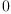(1) Type: Fraction(Polynomial(Fraction(Polynomial(Integer)))) fricas f(x) == (x^3+5*x-3)/(x^2-3) Type: Void fricas xlist := [1/2, 4, 1/6, 8, 1/10, 12]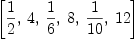(2) Type: List(Fraction(Integer)) fricas ylist := [f(x) for x in xlist] fricas Compiling function f with type Fraction(Integer) -> Fraction(Integer )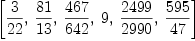(3) Type: List(Fraction(Integer)) fricas interpolate(xlist, ylist, 3, 2)$RINTERP('x, FRAC INT)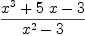(4)
Type: Fraction(Polynomial(Fraction(Integer)))
fricas
interpolate(1/6::FRAC UP(x,FRAC INT), xlist, ylist, 3, 2)$RINTERP('x,FRAC INT) The function interpolate is not implemented in RationalInterpolation (x,Fraction(Integer)) . A harder example: fricas dom := DMP([z],INT); Type: Type fricas g: FRAC dom -> FRAC dom; Type: Void fricas g(x) == (x^3*z+5*z^2*x -3*z^3)/(z*x^2 - 3) Type: Void fricas xxlist: List FRAC dom := [1/(2*z), 4*z, 1/(6*z), 8*z, 1/(10*z), 12*z]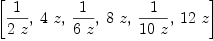(5) Type: List(Fraction(DistributedMultivariatePolynomial?([z],Integer))) fricas yylist := [g(x) for x in xxlist] fricas Compiling function g with type Fraction( DistributedMultivariatePolynomial([z],Integer)) -> Fraction( DistributedMultivariatePolynomial([z],Integer))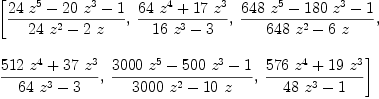(6) Type: List(Fraction(DistributedMultivariatePolynomial?([z],Integer))) fricas interpolate(xxlist, yylist, 3, 2)$RINTERP('x, FRAC dom)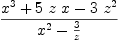(7)
Type: Fraction(Polynomial(Fraction(DistributedMultivariatePolynomial?([z],Integer))))
fricas
interpolate(4*z::FRAC UP(x,dom), xxlist, yylist, 3, 2)\$RINTERP('x, FRAC dom)
The function interpolate is not implemented in RationalInterpolation
(x,Fraction(DistributedMultivariatePolynomial([z],Integer))) .

 Subject:   Be Bold !! ( 14 subscribers )